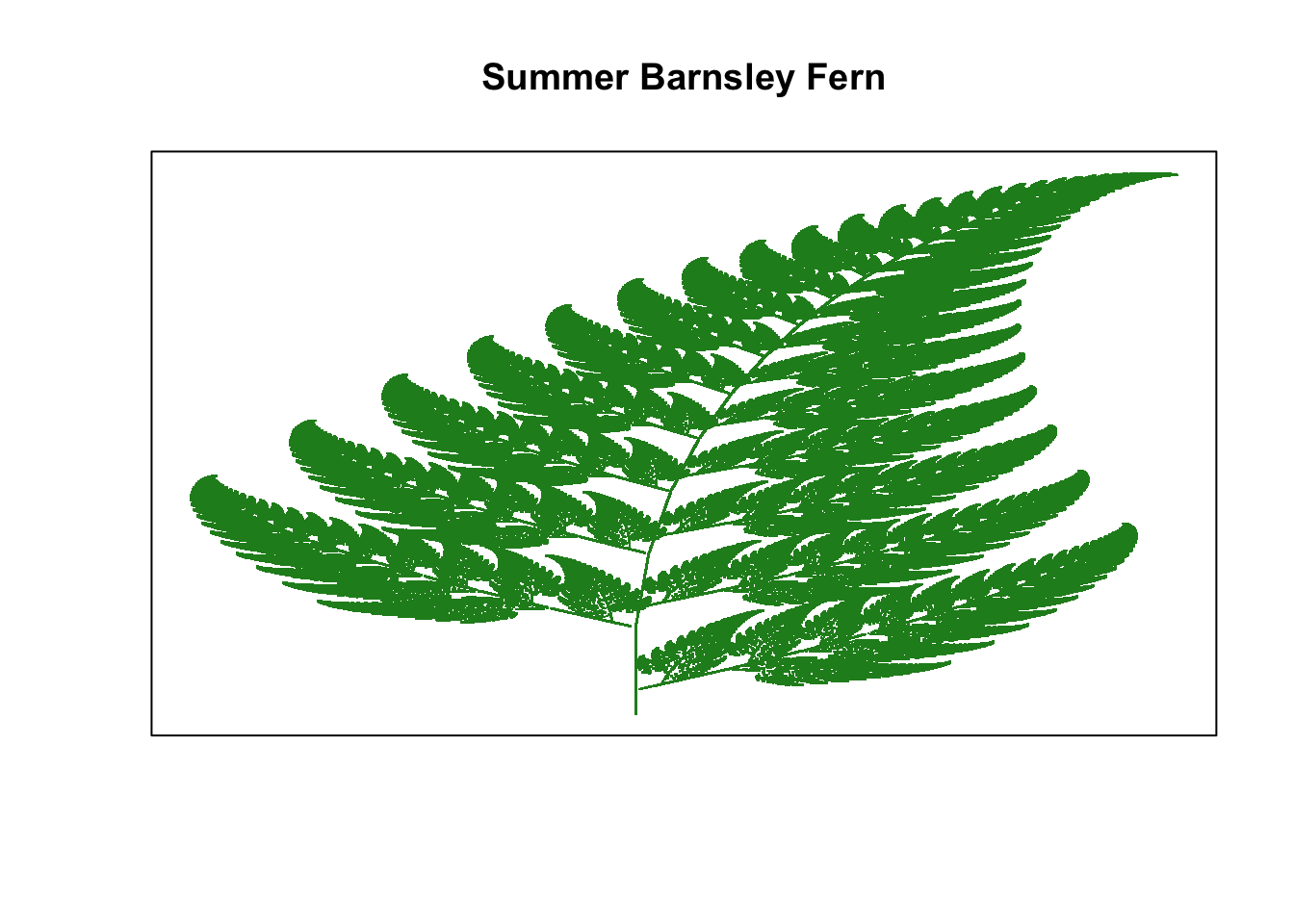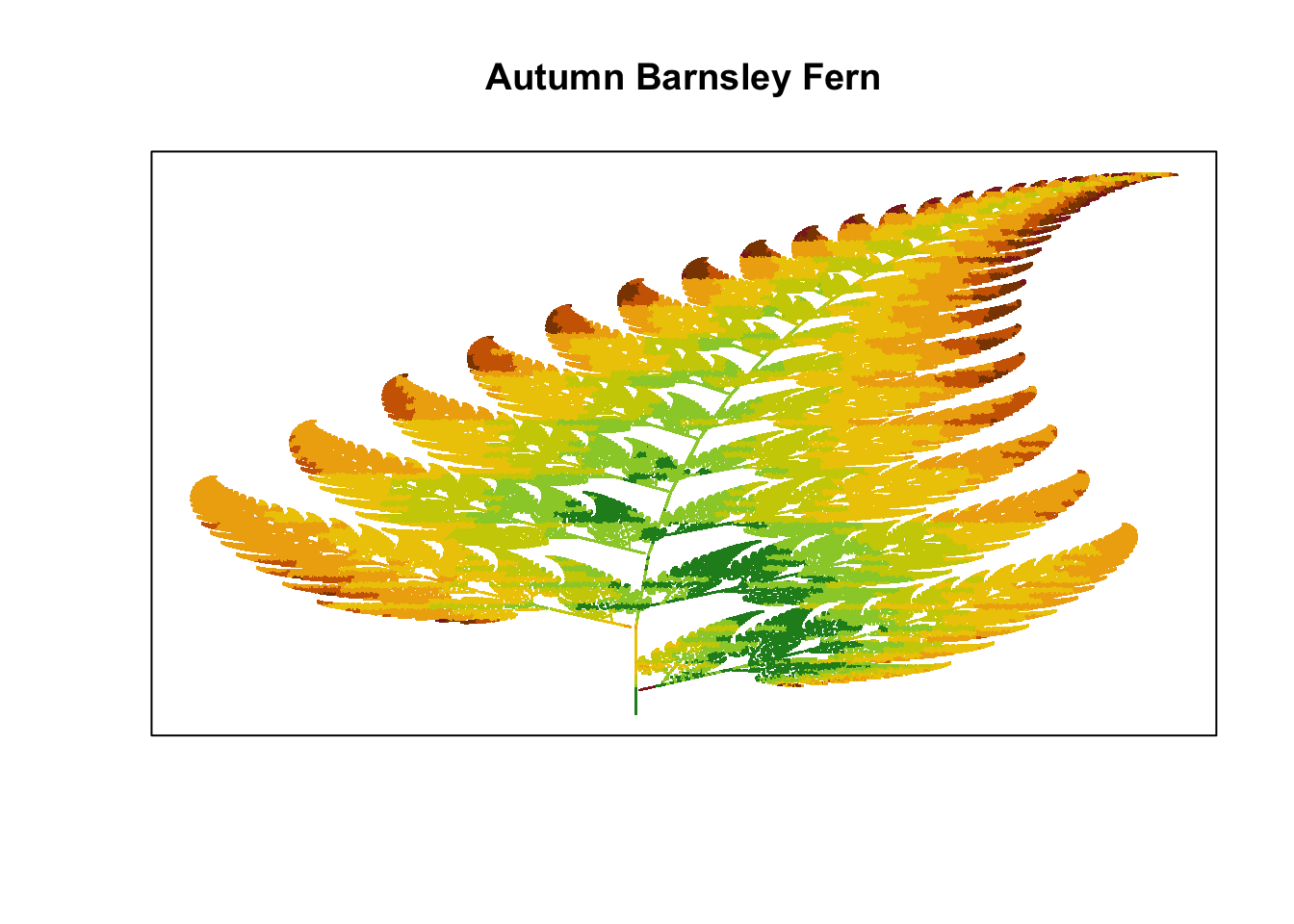# Autumn Barnsley Fern

stomperusa · 2019/10/13 · 3 minute read

### Intro

I was playing around generating fractals in R when I realized the monochromatic green Barnsely Fern I had on my screen didn’t quite look like the leaves I could see outside my window. It was already Fall. In this post I describe a technique to generate a Barnsley Fern with autumn foliage.

### The Barnsley Fern

First, we generate the Barnsley Fern.

#------------ Generate the Barnsely Fern ---------------

# Define the coefficients to calculate the x,y values

coef_names <- list(NULL,c("x","y","z"))

coef_x <- matrix(ncol = 3, byrow= TRUE, dimnames = coef_names,
c(0, 0, 0,
-0.15, 0.28, 0,
0.2, -0.26, 0,
0.85, 0.04, 0))

coef_y <- matrix(ncol = 3, byrow= TRUE, dimnames = coef_names,
c(0, 0.16, 0,
0.26, 0.24, 0.44,
0.23, 0.22, 1.6,
-0.04, 0.85, 1.6))

# Indicate the percentage of iterations through each row of the coefficients
coef_ctrl <- c(1,7,14,85)

# initialize a list to collect the generated points
points <- list()
points$x <- 0 points$y <- 0

# Set maximum iterations and reset coefficient tracker
max_i<-1000000
coef <- NA

# Generate the x,y points as a list and combine as a dataframe
for (i in 2:(max_i)) {
rand = runif(1, 0, 100)

if (rand < coef_ctrl){coef <- 1}
else if (rand < coef_ctrl){coef <- 2}
else if (rand < coef_ctrl){coef <- 3}
else {coef <- 4}

points$x[i] <- points$x[i-1]*coef_x[coef, 1] + points$y[i-1]*coef_x[coef, 2] + coef_x[coef, 3] points$y[i] <- points$x[i-1]*coef_y[coef, 1] + points$y[i-1]*coef_y[coef, 2] + coef_y[coef, 3]
}

df <- bind_rows(points)

We can plot the fern and to color it green is straight forward. We now have a Summer Barnsley Fern.

# Checkout your Summer Barnsley Fern
plot(df$x, df$y, pch = '.', col = "forestgreen", xaxt = "n", yaxt = "n", xlab = NA, ylab = NA,
main= "Summer Barnsley Fern")Now we consider the objective of coloring the leaf such that it reflects the patterns we see in the autumn. The edges of a leaf will usually change colors first so we want to have the red and organge tints on the sides of the leaf and yellow and green tints in the mid section along the stem. The leaf also tapers from bottom to tip and as the leaf thins at the top we expect more red and organge tints and less of the green.

To accomplish this, we color the fern in a way that is symmetrical and thus will base the color on the distance each x is from the mean of x coordinates. Because the fern curves as y increases we need to shift the colors to the right to follow the curve. This is accomplished by binning the y coordinates and calculating the mean of the x coordinates for each y bin.

df$ybin <- signif(df$y,2)

df <- df[-1,] %>%
group_by(ybin) %>%
mutate(sd = sd(x), mean = mean(x))  %>%
ungroup() %>%
mutate(xdev = abs(mean -x)/sd)

df[is.na(df$xdev),]$xdev <- 0

Because the fern also narrows at the top, we want to use proportionally more of the colors we use for the edges (farthest from the mean). Thus, we will factor in the value of y along with the x distance from the mean to determine the color for the point.

A histogram can help determine what break points to use for the colors.

#not run
#hist(df$xdev + (df$y/10))

We define our autumn color schematic and generate the Autumn Barnsley Fern.

# Set the breakpoints and the colors
color_table <- tibble( value = c(0.5, 0.8, 1.1, 1.5, 1.9, 2.1, 2.3, max(df$xdev + (df$y/10))),
color = c("forestgreen", "yellowgreen", "yellow3", "gold2",
"darkgoldenrod2", "darkorange3", "darkorange4", "brown4"))

# Lookup the corresponding color for each of the points.
df$col <- NA for (r in 1:nrow(color_table) ){ df$col[df$xdev + (df$y/10) <= color_table$value[r] & is.na(df$col)] <- color_table$color[r] } plot(df$x, df$y, pch = '.', col = df$col,xaxt = "n", yaxt = "n", xlab = NA, ylab = NA,
main = "Autumn Barnsley Fern")